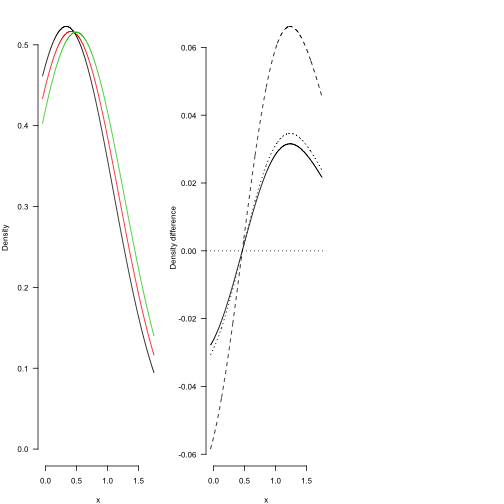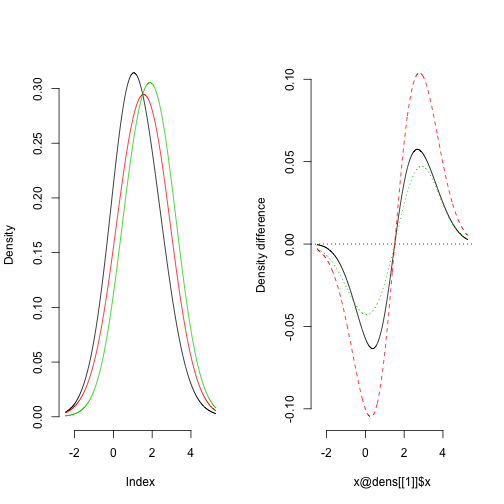## Appendix to “How to assess the existence of competing strategies in cognitive tasks: A primer on the fixed-point property”

Leendert van Maanen1, Ritske de Jong2, & Hedderik van Rijn2

1 University of Amsterdam, 2 University of Groningen

This documents illustrates how the fp package can be used. The fp package contains functions and helpful functions to assess the presence or absence of the fixed-point property in binary mixture distributions (Falmagne, 1968).

Here we document step by step the visualization and analysis of Simulation 1 in the manuscript. Simulation 1 illustrates the presence and absence of the fixed-point property in data that is or is not from binary mixture distributions.

### Steps to take for Simulation 1

First, load the required libraries. The fp package is required, and the SuppDists package, because it contains the Wald distribution function (invGauss).

``````require(fp)
require(SuppDists)
``````

Secondly, we generate data under the assumption that there is a fixed-point property. That is, we generate data for 50 participants in three mixture conditions (with mixture proportions p={.1, .5, .9}), with 200 observations per mixture condition. The parameters of the base distributions are mean = {.3,.5} and lambda = 5. For each participant a random intercept is added drawn from a normal distribution with mean 0 and standard deviation .1

``````
N <- 200  # nr of observations per condition
M <- 50  # nr of participants
p <- seq(0.1, 0.9, 0.4)  # mixture proportions
means <- c(0.3, 0.5)  # means of base distributions
lambda <- 5  # scale of base distributions

### generate data
rt <- NULL
for (i in 1:length(p)) {
rt <- c(rt, ifelse(sample(0:1, N * M, replace = T, prob = c(p[i], 1 - p[i])),
rinvGauss(N * M, means, lambda), rinvGauss(N * M, means, lambda)))
}
rt <- rt + rep(rnorm(M, sd = 0.1), times = N)  # normally distributed pp random effect
dat <- data.frame(rt = rt, cond = rep(1:length(p), each = N * M), pp = rep(1:M,
each = N))
``````

We can visualize the fixed-point property by plotting the density functions of each condition as well as the differences between the densities. This can be achieved by calling plot or plot.fp1 on an fp1 object, which contains the density and difference information. The function fpGet provides an fp1 object based in a standard dataframe with either two (response time and condition) or three (response, time, condition, and participant number) columns. The smoothing bandwidth as well as the number of points for which the density is computed can be set, but these do not influence the results (see Simulations 2 and 3 in the main text).

``````res <- fpGet(dat[, c("rt", "cond")], 1000, bw = 0.75)  # get the fp1 object
par(las = 1, mfrow = c(1, 3), mar = c(4, 4, 1, 1))  # make sure the plots are side by side
plot(res, xlab = "x", col = 1)  # call plot
``````To provide statistical evidence in favor of the presence of the fixed-point property, we can call fpAnova. fpAnova takes two arguments, a list of fp1 objects (one for each participant) and a specification of which tests to perform (p, BF, or both).

``````res <- tapply(1:nrow(dat), dat\$pp, function(X) {
fpGet(dat[X, ], 1000, bw = 0.75)
})  # compute the list
fpAnova(res, stat = "both")
``````
``````## \$BF
## Bayes factor analysis
## --------------
##  cross + pp : 0.07086 <U+00B1>1.02%
##
## Against denominator:
##   x ~ pp
## ---
## Bayes factor type: BFlinearModel, JZS
##
##
## \$p
##
## Error: pp
##           Df Sum Sq  Mean Sq F value Pr(>F)
## Residuals 49 0.0322 0.000657
##
## Error: pp:cross
##           Df Sum Sq  Mean Sq F value Pr(>F)
## cross      2 0.0002 0.000078    0.09   0.92
## Residuals 98 0.0892 0.000911
``````

A Bayes factor below 1 generally means support for the null hypothesis. To see how much more likely the null hypothesis is than the alternative hypothesis, one has to take 1/BF. We refer to Kass & Raftery (1995) for qualifications of the different Bayes factors. In addition, a high p-value (often higher than .05) is considered to provide no reason to reject the null hypothesis, which is consistent with the presence of the fixed-point property.

The effect can be visualized by showing a boxplot of the crossing points per condition pair.

``````crosses = fpDensDiff(res)  # first, get the crossing points
boxplot(t(crosses), frame.plot = F, xlab = "Crossing point", ylab = "Condition pair",
names = c("1-2", "2-3", "1-3"), horizontal = T)
``````examples used in the fp package

``````## generate data
p <- c(0.1, 0.5, 0.9)
rt <- sapply(1:3, function(i) {
rnormMix(1000, c(1, 2), c(1, 1), p[i])
})
dat <- data.frame(rt = c(rt), cond = rep(1:3, each = 1000))
fpobject <- fpGet(dat, 1000, bw = 0.75)
par(mfrow = c(1, 2))
plot(fpobject)
````````````## generate data
p <- c(0.1, 0.5, 0.9)
rt <- sapply(1:3, function(i) {
rnormMix(10000, c(1, 2), c(1, 1), p[i])
})
dat <- data.frame(rt = c(rt), cond = rep(1:3, each = 10000), pp = rep(1:50,
each = 200, times = 3))
## compute the list of fp objects
res <- tapply(1:nrow(dat), dat\$pp, function(X) {
fpGet(dat[X, ], 1000, bw = 0.75)
})
## call fpAnova, with stat='both' to do both a Bayesian and a frequentist
## test
fpAnova(res, stat = "both")
``````
``````## \$BF
## Bayes factor analysis
## --------------
##  cross + pp : 0.1465 <U+00B1>0.62%
##
## Against denominator:
##   x ~ pp
## ---
## Bayes factor type: BFlinearModel, JZS
##
##
## \$p
##
## Error: pp
##           Df Sum Sq Mean Sq F value Pr(>F)
## Residuals 49   1.52  0.0309
##
## Error: pp:cross
##           Df Sum Sq Mean Sq F value Pr(>F)
## cross      2  0.047  0.0233    0.87   0.42
## Residuals 98  2.640  0.0269
``````
``````## generate data
p <- c(0.1, 0.5, 0.9)
rt <- sapply(1:3, function(i) {
rnormMix(10000, c(1, 2), c(1, 1), p[i])
})
dat <- data.frame(rt = c(rt), cond = rep(1:3, each = 10000), pp = rep(1:50,
each = 200, times = 3))

## compute the list of fp objects
res <- tapply(1:nrow(dat), dat\$pp, function(X) {
fpGet(dat[X, ], 1000, bw = 0.75)
})

## get the crossing points
crosses = fpDensDiff(res)
boxplot(t(crosses), frame.plot = F, xlab = "Crossing point", ylab = "Condition pair",
names = c("1-2", "2-3", "1-3"), horizontal = T)
``````